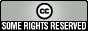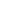Finite Geometry Notes   | Home | Site Map | Author |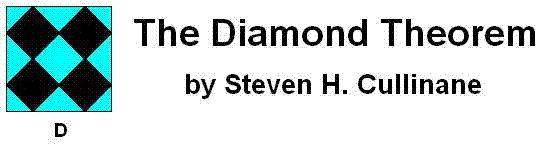ABSTRACT: Finite projective geometry explains the surprising symmetry properties of some simple graphic designs-- found, for instance, in quilts. Links are provided for applications to sporadic simple groups (via the "Miracle Octad Generator" of R. T. Curtis), to the connection between orthogonal Latin squares and projective spreads, and to symmetry of Walsh functions.
We regard the four-diamond figure D above as a 4x4 array of two-color diagonally-divided square tiles.

Let G be the group of 322,560 permutations of these 16 tiles generated by arbitrarily mixing random permutations of rows and of columns with random permutations of the four 2x2 quadrants.

THEOREM: Every G-image of D (as at right, below) has some ordinary or color-interchange symmetry.

Example: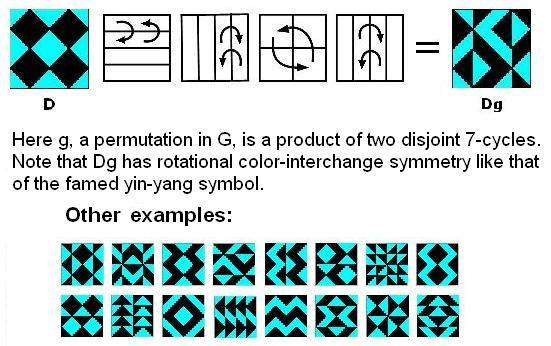For an animated version, click here. Remarks: Some of the patterns resulting from the action of G on D have been known for thousands of years. (See Jablan, Symmetry and Ornament, Ch. 2.6.) It is perhaps surprising that the patterns' interrelationships and symmetries can be explained fully only by using mathematics discovered just recently (relative to the patterns' age)-- in particular, the theory of automorphism groups of finite geometries. Using this theory, we can summarize the patterns' properties by saying that G is isomorphic to the affine group A on the linear 4-space over GF(2) and that the 35 structures of the 840 = 35 x 24 G-images of D are isomorphic to the 35 lines in the 3-dimensional projective space over GF(2). This can be seen by viewing the 35 structures as three-sets of line diagrams, based on the three partitions of the four-set of square two-color tiles into two two-sets, and indicating the locations of these two-sets of tiles within the 4x4 patterns. The lines of the line diagrams may be added in a binary fashion (i.e., 1+1=0). Each three-set of line diagrams sums to zero-- i.e., each diagram in a three-set is the binary sum of the other two diagrams in the set. Thus, the 35 three-sets of line diagrams correspond to the 35 three-point lines of the finite projective 3-space PG(3,2). For example, here are the line diagrams for the figures above: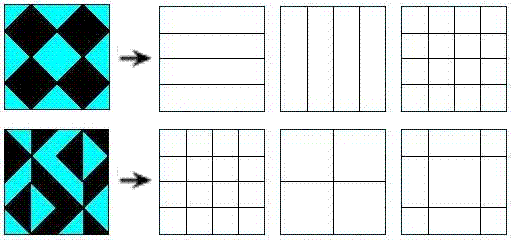Shown below are the 15 possible line diagrams resulting from row/column/quadrant permutations. These 15 diagrams may, as noted above, be regarded as the 15 points of the projective 3-space PG(3,2).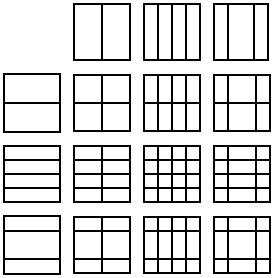The symmetry of the line diagrams accounts for the symmetry of the two-color patterns. (A proof shows that a 2nx2n two-color triangular half-squares pattern with such line diagrams must have a 2x2 center with a symmetry, and that this symmetry must be shared by the entire pattern.)

Among the 35 structures of the 840 4x4 arrays of tiles, orthogonality (in the sense of Latin-square orthogonality) corresponds to skewness of lines in the finite projective space PG(3,2). This was stated by the author in a 1978 note. (The note apparently had little effect. A quarter-century later, P. Govaerts, D. Jungnickel, L. Storme, and J. A. Thas wrote that skew (i.e., nonintersecting) lines in a projective space seem "at first sight not at all related" to orthogonal Latin squares.)

We can define sums and products so that the G-images of D generate an ideal (1024 patterns characterized by all horizontal or vertical "cuts" being uninterrupted) of a ring of 4096 symmetric patterns. There is an infinite family of such "diamond" rings, isomorphic to rings of matrices over GF(4).

The proof uses a decomposition technique for functions into a finite field that might be of more general use.

The underlying geometry of the 4x4 patterns is closely related to the Miracle Octad Generator of R. T. Curtis-- used in the construction of the Steiner system S(5,8,24)-- and hence is also related to the Leech lattice, which, as Walter Feit has remarked, "is a blown up version of S(5,8,24)."

For movable JavaScript versions of these 4x4 patterns, see The Diamond 16 Puzzle and the easier Kaleidoscope Puzzle.

The above is an expanded version of Abstract 79T-A37, "Symmetry invariance in a diamond ring," by Steven H. Cullinane, Notices of the American Mathematical Society, February 1979, pages A-193, 194.

For some context, see Reflection Groups in Finite Geometry.

Related pages:

The Diamond 16 Puzzle

Diamond Theory in 1937:
A Brief Historical Note

Notes on Finite Geometry

Geometry of the 4x4 Square

The Geometry of Qubits

The Geometry of Logic

Binary Coordinate Systems

The 35 Lines of PG(3,2)

Map Systems:
Function Decomposition over a Finite Field

The Diamond Theorem--
The 2x2, the 2x2x2, the 4x4, and the 4x4x4 Cases

Diamond Theory

Latin-Square Geometry

Walsh Functions

Inscapes

The Diamond Theory of Truth

Geometry of the I Ching

Solomon's Cube and The Eightfold Way

Crystal and Dragon in Diamond Theory

The Form, the Pattern

The Grid of Time

Block Designs

Finite Relativity

Theme and Variations

Models of Finite Geometries

Quilt Geometry

Pattern Groups

The Fano Plane Revisualized,
or the Eightfold Cube

Kaleidoscope

Visualizing GL(2,p)

Jung's Imago